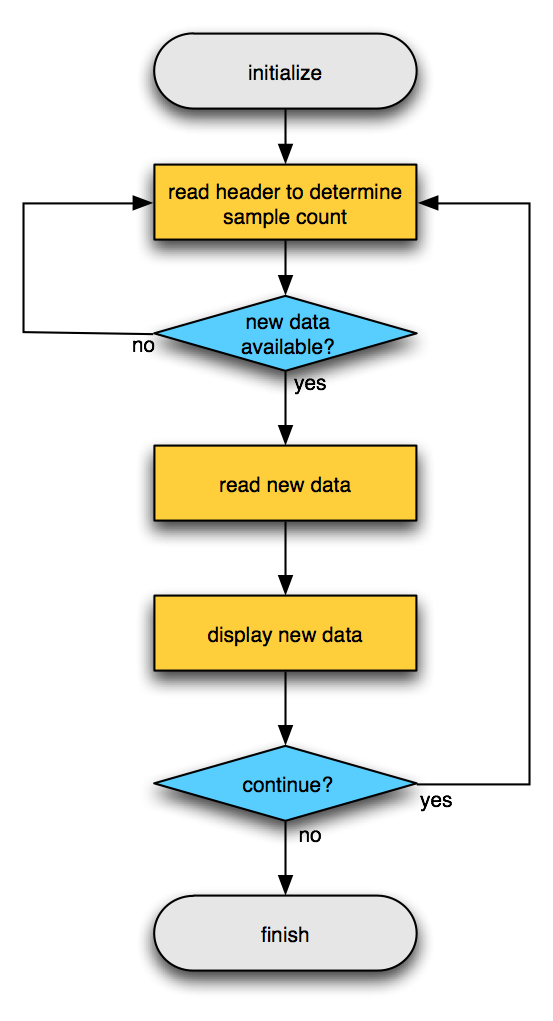Tags: example realtime

# Example real-time signal viewer

The simplest example for continuous data without any events or triggers is a simple data viewer that lets the data scroll by in a figure, while the data streams from the acquisition system. This example is implemented in the ft_realtime_signalviewer function. You can of course take this function as starting point for your BCI system and modify it to your own needs.

## Flowchart## Example use

The easiest way to try out the ft_realtime_signalviewer example is by starting two MATLAB sessions. In the first session you create some random signal and write it to the buffer.

``````cfg                = [];
cfg.channel        = 1:10;                         % list with channel "names"
cfg.blocksize      = 1;                            % seconds
cfg.fsample        = 250;                          % sampling frequency
cfg.target.dataset = 'buffer://localhost:1972';    % where to write the data
ft_realtime_signalproxy(cfg)
``````

In the second MATLAB session you start the ft_realtime_signalviewer and point it to the buffer.

``````cfg                = [];
cfg.blocksize      = 1;                            % seconds
cfg.dataset        = 'buffer://localhost:1972';    % where to read the data
ft_realtime_signalviewer(cfg)
``````

After starting the ft_realtime_signalviewer, you should see a figure that updates itself every second. That figure contains the raw signal. You can also start the two MATLAB sessions on two different computers, where on the second you would then point the reading function to the first computer.

## MATLAB code

The code for ft_realtime_signalviewer is included in the FieldTrip release under `fieldtrip/realtime/example` and can also be found on GitHub.